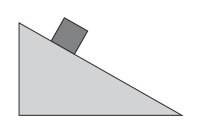# Block on a Ramp#

A mass of 41 $$kg$$ sits at rest on an incline making an angle of 16 $$^\circ$$ with respect to the horizontal.## Part 1#

If $$\mu_s$$ = 0.9, what is the friction force on the block? Choose the best answer.

• 110.0 $$N$$, up the incline

• 390.0 $$N$$, up the incline

• 100.0 $$N$$, down the incline

• 350.0 $$N$$, down the incline

• 110.0 $$N$$, down the incline